# An introduction to CFD

Engineers predict the behaviour of things on a regular basis. “Will this bridge hold under load? Will it hold in high winds? Are there any unexpected structural harmonics that might lead to undesirable instability?” are examples of the kind of prediction-seeking questions that engineers ask on a regular basis.

One of the methods that engineers use to answer these questions is a very helpful tool called computational fluid dynamics (CFD for short), a technique for determining the behaviours and dynamics of fluids. This is a category that includes both liquids and gases but, under the right conditions, almost anything can flow like a fluid.

The need to understand and manipulate fluids touches almost every part of our lives, and CFD is correspondingly present in many fields of industrial design. From more fuel-efficient cars to laptop cooling systems, aircraft dynamics to air conditioning, vast amounts of computational effort are expended in the interests of keeping us safer, wealthier, and at a comfortable temperature. But how does this tool work? And crucially, can we make it work better?

Like many other tools in recent years, CFD can benefit from the ongoing revolution in computing. From new techniques to better hardware, a silent revolution is occurring that might sweep away huge sections of what was once considered to be the conceptual core of CFD in favour of something quite different indeed.

In this article, we aim to provide an illustration of where the field is now so that we can later talk in detail about where it might end up in the future. If you’re in a hurry, there’s a summary you can skip to at the end of this article. If not, we start by describing a method of making approximations for complex problems. We then describe what the problems are with that method and move on the specific techniques for performing CFD. We illustrate how the most common CFD technique works and some of the more important issues with it, and explain why it might be time for another method. We finish off by describing what these methods could be.

### Making predictions for complex mechanical problems

Rather than leaping straight in to describing the fundamentals of CFD, we’ll start with a closely related approach in terms of the mechanics of solid materials. Making predictions is relatively straightforward when working with solid materials, because by definition they obey certain structural relationships. In many of the problems that we’d like to find solutions for, each “bit” of a solid material remains in roughly the same position relative to every other “bit” over time, making it easier to construct and solve problems.

### Big solutions from smaller problems

Take the following example of a metal beam, supported at both ends, being subjected to a downwards vertical force.If the force is big enough, the beam will bendFinding the force needed to cause the beam to bend is a matter of knowing the yield conditions of the material and working out moments. What is less easy to work out is the exact shape that the beam will take after being bent. Fortunately, as we said earlier, each bit of a solid material tends to retain an overall consistent position with respect to each other bit.

This allows us to use a very useful technique in which we can discretise (break up) the beam into smaller pieces and then solve the equations of motion for each discrete “bit” or element. Here’s what it looks like when we divide the beam up into smaller pieces, which we collectively call a grid or mesh:In this method, each element is completely filled with a fixed piece of the solid; no other material can enter the element, and no material leaves the element. However, the mesh elements can change shape in response to forces. If it helps, think of the beam as being made of really hard bits of blu-tack which are firmly stuck together. How strongly these pieces are stuck together and how they deform in response to forces is described by the material model, a collection of one or more mathematical relationships that tie these things together.

When we apply the same force as before, we now have a number of individual equations to solve across all the different bits of the beam; these will all have different solutions depending on where each element is on the beam:Each element of the mesh now experiences different forces, resulting in different motions

And the final shape of the beam is given by the cumulative solutions to the motion of each individual element:This gets us something close to the “correct” final shape of the beam, the shape that we would get if we did the same thing in real life (assuming that our simulation captured the relevant physical laws), but this is a tremendous amount of extra work! For a very basic 2-dimensional problem like the one above, solving all of the relevant equations by hand would take hours and be extremely repetitive. For larger and more complex geometries in 3D, it almost certainly wouldn’t be worth it.

### Timestepping

To make matters worse, we can’t perform the solution in one go; to accurately reach the final state of the beam means that we have to take small steps in time as well as space.We can’t simply jump to the end solution; just like in real life, we have to move forwards through time. There are two distinctly different time-stepping techniques known as “implicit” and “explicit” methods. Implicit methods allow for larger time-steps but struggle with discontinuities (such as shockwaves), while explicit methods can capture shocks and other very fast events at the cost of much shorter timesteps.

While there are two different approaches to time-stepping, the general principle is the same; solutions of the system at earlier time-steps are used when calculating a solution over the next time-step. This is true of implicit methods (where the calculations also involve unknown or “implicit” future values) and explicit methods (where only currently-known or “explicit”values are used to calculate the next set of values).

Breaking the problem down into tiny pieces and timesteps means solving a phenomenal number of equations, but being able to predict these behaviours ahead of time is incredibly useful; not only is it cheaper and faster than repeatedly building physical prototypes, but in some cases, such as the design of large buildings or aircraft, many lives can depend on it. We therefore do what we always do when faced with a big, tedious task:

### We do it all with computers

The computational load for working out a small problem like the one described above is still relatively large. The average laptop would probably take a minute or so to calculate the solution, assuming we wanted it to be physically accurate rather than just visually so. This isn’t a very long time, but it does give some indication of how much computing needs to be done. After all, in our daily lives, we’re used to computers completing “small” tasks more or less immediately.

When we scale this up to millions of elements (easily done, especially if we’re solving the problem in 3D), the amount of computation that needs to be performed increases drastically. Large-scale simulations are therefore almost exclusively the preserve of high-performance computing, in which hundreds or even thousands of processing cores work simultaneously on the solution.

This is expensive in three different ways; money, time and energy. First, you either need to buy and maintain a supercomputer, or at least rent the use of one. You’ll also need to pay for licenses for most engineering software packages.

Second, even with sufficient computing power, these techniques take a lot of time, because the timesteps have to be calculated in sequence. There’s also diminishing returns to be had from using more and more cores, a phenomenon known as Amdahl’s law. It’s therefore not unusual for large simulations to take weeks to run even on a powerful supercomputer.

Third, running these systems wastes a vast amount of energy. If you’re only interested in the final state of the solution, you still have to solve a significantly greater number of calculations getting to that final point, because you have to work your way forward through all the time-steps that lie between the start of the simulation and the end point. It’s very rare that you would need all of the data produced throughout a simulation, even if you want to investigate the dynamics over time. If you did want it, saving all the data produced by a very big simulation would require staggering amounts of storage space.

We’ll leave these issues for now, but it’s worth remembering them; these considerations will come up later when we start thinking about better methods.

### Basic computational methods for fluid simulation

The method we described above for working out the deformation of the beam is known as the finite element method. The “finite” bit refers to the fact that the individual elements have a defined size. This is different to the way in which the mathematics we usually describe these problems with (calculus) deals with infinitesimally small elements.A comparison of the concept of a finite element, which has a defined side length, compared to the limit-based concept of an infinitesimal element as used in calculus

This introduces sources of error into our simulation because we’re now making a numerical approximation to the behaviour of the analytic solution.A comparison between some analytic function f(x), an approximation to it using finite differences, and what happens as we start to approach an infinitesimal difference. The difference between the approximation and the analytic function (highlighted in green) is an error. As the size of the difference approaches an infinitesimal value, the “fit” of the approximation is better, until eventually we return to the analytic function in a way that can be worked on using calculus.

Some of this error is inescapable, because we need to break the problem up to work on it with a computer, and computers can only hold a limited number of values in memory.

Thus far we haven’t delved too deep into any mathematics because the finite element method isn’t a good method for working with fluids, although it is a good starting point for the general idea.

Solids (at least up to certain physical limits) obey relationships that mean we can break them down into individual elements that have their own dynamics. What about fluids? What happens when we try to solve fluid motion in the same way as we did the solid bar?

If we tried to treat a fluid in the same way, we’d start by dividing the fluid up into individual elements just as we did before. Each one of these elements contains a fixed amount of fluid; no other fluid can enter the element, and none can leave. Just like in the solid example above, if the element is subjected to forces, the shape of the element changes.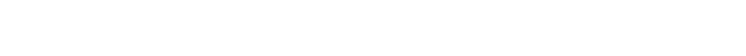A simple fluid-carrying pipe containing a small obstruction, a very basic (yet non-trivial) fluid flow environment. The fluid moves from left to right with a consistent flow rate, where more material is added at the leftmost inlet and is removed from the simulation at the outlet.

You can probably see that this is going to get extremely messy very quickly, because fluids do not work in the same way as solids; they can slide past each other, swirl around and so on. Fluids aren’t connected or constrained in the same way that elements in a solid body are.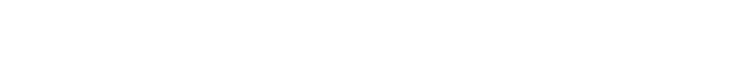An attempt to solve a fluid problem using the same method as for a solid body is doomed to rapidly experience what is known as “mesh tangling”; even the best solver packages will quickly fail due to extreme deformation and limits on numerical precision. Elements are coloured by velocity.

This approach using a connected mesh fails because of the incredible amounts of deformation a fluid element can undergo. But what if, instead of preserving the contents of each element and deforming the mesh, we do things the other way around; we keep the mesh shape consistent and allowing the contents to move between elements?Much better! Although in practice the size of the mesh in the picture is much larger than we would normally use.

This kind of mesh, in which the structure of the mesh is fixed and material moves between elements, is known as an Eulerian mesh. The other kind, where material is embedded in the mesh and the mesh deforms with the material, is known as a Lagrangian mesh.

These two approaches are the most common solutions* for solving material simulation problems, although they aren’t the only methods. Some approaches, such as smooth-particle hydrodynamics, do away with meshes entirely. Picking the right technique for a problem isn’t always easy; it’s very dependent on the problem and the materials, but for most engineering problems in fluid dynamics the Eulerian approach is extremely popular.

However, thus far we’ve yet to answer an important question; what exactly is it that we are transporting between cells?

*(Despite their differences, Lagrangian and Eulerian meshes can also work together in the same simulation; in some cases, we can even switch between them on the fly. This is the basis of many approaches to solving fluid-structure interactions)

### The Navier-Stokes equations

Fluid motion is famous for being complex, but the mathematical treatment of it arises from some very basic principles. Mass, momentum and energy have to be conserved; simply put, in any physical process, you can’t end up with more (or less!) matter and energy than you started with. Laws that reflect this fact are therefore known as conservation laws.

This section is more mathematically complex; if you’re not interested in these details, skip ahead to the next section, which deals with one of the biggest problems in fluid dynamics. For now, we can just say that we use a few mathematical tools to turn the laws of fluid motion into many smaller but simpler equations that allow us to calculate the transport of fluid properties (mass, momentum, energy and so on) between individual fluid volumes.

. . .

The most complete version of the conservation laws for fluids are known as the Navier Stokes equations for compressible, viscid flow. This is a mathematical model that accounts for the full range of conditions that can occur within a fluid.These are the Navier Stokes equations, written in what is known as the “conservation” form most usually associated with the Eulerian method outlined above. There’s no single definitive notation for these equations, so you may see them written differently elsewhere depending on how they are being used.

Despite their complexity, the general thrust of the Navier Stokes equations can be quite readily understood. We won’t go into exhaustive detail, but it’s helpful to understand that in each equation, the similar-looking termsall represent the divergence (the net inflow or outflow) of mass, momentum and total energy within the volume.

In the mass equation, the change in fluid density is directly related to the total mass flowing into and out of the volume. In the momentum equations, the bulk change in momentum is related to the different forces that can act on the fluid volume. The energy equation associates the bulk change in energy with changes in the sum of kinetic, potential and thermal energies in the volume.

The physical origins of the equations might be readily explicable, but the way they behave are not. From the perspective of the fundamental mathematics, the Navier-Stokes equations are a set of partial differential equations for which there is currently no analytic solution for most starting configurations!

In fact, simply finding a formal definition of the global solution space of the Navier-Stokes equations is one of the Clay Mathematics Institute Millenium Prize problems. There’s an associated prize of \$1,000,000 if you can find an analytic method for mapping the velocity field of a fluid to the associated pressure field.

Fortunately, our approximation method means that we aren’t reliant on there being an analytic solution. However, we still need a way of turning these partial differential equations into something that a computer can work with.

There’s a very useful tool that allows us to relate the divergence of properties contained within the volume to the flow or “flux” of these values through the surface. It’s called Gauss’s theorem.Gauss’s theorem illustrated visually. The black arrows represent the divergence of a quantity contained within the blue cube. The red dots on the cube’s surface indicate where those lines of divergence pass through the surface of the volume. Drawn this way, we can see how divergence and the flux through a surface are related.The mathematical interpretation of Gauss’s theorem. The volume integral of the divergence of a field is equivalent to the surface integral of the scalar product of the field and the surface normal.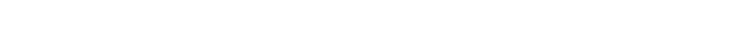Where the terms in bold represent vectors columns of flow quantities, broken up into scalar quantities WVector quantities F, GAnd body forcesFrom these individually simpler representations, we can begin constructing algorithms for transporting flow quantities at the interfaces between cells.An illustration of the net direction of the transport of a fluid property (e.g mass) between Eulerian mesh cells in a 2-dimensional region. When calculated for all the relevant properties, the resulting changes across the flow domain should describe the associated fluid dynamics, just as solving the equations of motion for individual elements does for the solid body in the earlier example. The total value of these properties is conserved in accordance with the conservation laws that we used to derive the transport equations.

Because we have broken these quantities up into different vectors, this also means that we can apply different mathematical techniques to each, allowing us to use more carefully tailored calculation methods that yield more accurate results.

Eulerian meshes lie at the heart of most approaches to CFD, and flux transport schemes are what make them tick. However, there’s now another problem heading towards us, one that comes from this blending of meshes with fluid transport and threatens to stop us in our tracks if we try and do anything complicated.

It’s time to talk about turbulence.

### Turbulence

“When I meet God, I am going to ask him two questions: Why relativity? And why turbulence? I really believe he will have an answer for the first one” — Apocryphal, attributed to Werner Heisenberg

Turbulence is the unpredictable motion of a fluid. While it might be tempting to try and give a more “complete” definition, the truth is that the exact origins and behaviour of turbulence are still an unsolved problem.

However, there are some things that we can say about turbulence. Amongst other things, it is fundamentally chaotic and it is very hard to work out turbulent motion from first principles, even though the Navier-Stokes equations notionally give us everything we need to do so.

Secondly, turbulence is strongly associated with rotation. Turbulence manifests as swirling, rotating eddies or vortices in the flow, regions where the fluid isn’t flowing in a straight line but is instead rotating and mixing. Because the fluid now contains many of these rotating eddies, the velocity at any one point in a turbulent flow will fluctuate wildly.

This rotation is opposed by the viscosity of the fluid, a measure of how strongly it resists deformation. As an example, you can put your hand in a bucket of water and freely swirl it around, whereas this is somewhat harder to do if the bucket is full of syrup. Both are fluids, but the syrup is much more viscous.

Viscosity can be thought of as arising from the friction between fluid elements, which in turn introduces the idea of fluid stresses. These are represented in the Navier-Stokes equations as the terms containing 𝜏, with the interpretation of how these stresses interact with the surface of a fluid volume shown below:An infinitesimally small volume of fluid and the stresses that can act on the faces. The two stresses that lie in the same plane as each face are shear stresses. If you’ve ever used a pencil eraser to rub something out, the little sausage-shaped flecks left behind are the result of shear stresses and their tendency to rotate deformable materials.

So there’s a link between turbulence and fluid stress. It isn’t the whole story, but it’s a good place to start.Simplified view of the flow over a solid wall. The fluid in direct contact with the wall has no velocity at all. This creates friction with the layer of fluid directly above, which moves slowly. The layer above this experiences less friction and moves a little quicker, and so on until the flow reaches a “free stream” condition. In this case, each incredibly thin layer is moving in the same direction in a “laminar” flow.The velocity difference between layers (black arrows) causes “inertial” shear-stress forces (red arrows) to act on a small fluid element which is moving with the flow.In a laminar flow, the viscous forces (blue arrows) resist deformation; the fluid element keeps going in a straight line in a laminar flow.If the ratio of inertial forces and viscous forces is not in balance, the fluid will also experience a net rotation or “vorticity”. This is the origin of “shear-induced” turbulence.

This relationship between the inertial forces and viscosity gives us a way of predicting the onset of turbulence through the Reynolds number, a dimensionless value that takes into account both the basic fluid properties and the scale of the flow.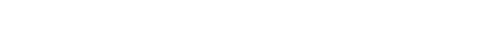The Reynolds number; ρ is the fluid density, u is the velocity, L is the “characteristic lengthscale” of the flow and μ is a measure of the fluid viscosity (in this example, specifically the “dynamic viscosity”)

If this ratio is small then the fluid moves as a laminar flow; however, above a “critical value” the forces start to become unbalanced and the flow quickly transitions into a turbulent flow.

Rotating flows also represent a velocity gradient, so larger vortices tend to “shed” smaller vortices, which in turn shed even smaller vortices! This process transfers rotational kinetic energy from the largest turbulent length-scales in the flow down to the smallest “dissipative” length-scales, and only ends when the vortices become small enough that the viscous and inertial forces are once again in balance.This “energy cascade” means that to properly maintain the continuity of energy in a simulation, we need to be able to capture the smallest rotational features of the flow. This sets a very small maximum size for our computational volumes, which in turn drastically increases the number of calculations we need to perform.

So how small do we need to go? We won’t go deep into the specifics, but for a 3 dimensional simulation the number of volume elements needed to capture the full turbulent cascade scales as the Reynolds number to the power of 2.25.

For a 20mm wide pipe containing water moving at 0.2 meters per second, the Reynolds number is ~4481. Resolving the turbulent flow within a volume of around 20mm³ would require approximately 164,275,036individual volumes!

To make matters worse, the maximum (explicit) timestep that can be applied is limited by the grid scale. In explicit time-stepping, we can’t have a time-step where the distance travelled by the fluid could be greater than the smallest grid element; information from one volume can only travel to its immediate neighbours in a given timestep. This is known as the Courant stability conditionwhere C must less than or equal to one.Illustration of the difference between a timestep that doesn’t breach the Courant stability criteria and one that does. In the first case (C<1), all the information from the leftmost cell is transferred to its neighbour on the right. In the second case, (C>1), this information has essentially “skipped” the middle cell, a non-physical violation of causality.

From the grid sizing, the maximum timestep we could use would be around~18 microseconds; solving the flow over a period of just one second would therefore require us to solve the transport equations for those 164,275,036 individual volumes 55,555 times, or about 9 trillion complete sets of transport equations in total. All for a tiny section of pipe containing a very common fluid moving at relatively slow speeds.

This is still achievable with modern computers, but it is incredibly expensive and time consuming. If we’re going to use CFD for more complex flows and higher Reynolds numbers, we need something more efficient.

### The part where it gets complicated

Until now, everything was working out. We had a method for managing big problems by breaking them down into smaller ones, which works very well for solid mechanics. We were able to adapt this to fluid dynamics by changing the way we thought about the mesh and work out the starting points for the transport equations between volumes.

Turbulence, and the tiny control volumes that are required to resolve it, introduces a problem which is not tractable for many fluid dynamics problems even in an age of extremely fast large-scale computing. Yet turbulence is vital to understanding fluid flow and making predictions using CFD, so how have we been doing it so far?

In some cases, we simply resign ourselves to the computational expense and directly resolve the turbulent lengthscales. You can see the outcome (and the incredible detail) of a 2-dimensional DNS simulation in the video below.

A direct numerical simulation of the fluid flow around a square in 2 dimensions. As time passes, the separation point of the flow moves up and down, creating a periodic structure in the downstream flow known as a Von Karman street

However, for most practical purposes, we can also treat turbulence as a mathematical model, without having to provide enough spatial resolution to resolve the individual eddies.

### Turbulence as a mathematical model

Turbulent flows are chaotic, which means that we can treat turbulence as a statistical phenomenon, allowing us to split the velocity field of a flow into a mean velocity (representing the overall bulk movement of the flow) and a fluctuating velocity that represents the statistical fluctuations of turbulence. Substituting this change in the representation of the velocity into the Navier-Stokes equations gives us the Reynolds-averaged Navier-Stokes (RANS) approach, which accounts for the bulk of practical “engineering” CFD simulations.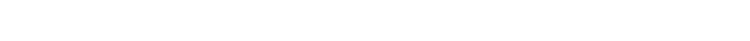The momentum equation in the Reynolds-averaging approach. The values of velocity followed by a dash are the fluctuating velocity components, while the over-bar indicates the mean value over time.

Reynolds averaging leaves us with Reynolds stresses which relate the velocity fluctuations to the stress terms in the original Navier-Stokes equationsWe now have some new values that we need to calculate in order to close the system of equations, so we need a way of calculating these Reynolds stresses. For “Newtonian” fluids (which includes things like air and water), the viscous stresses can be assumed to be proportional to the mean velocity gradient. This assumption is known as the Boussinesq hypothesis: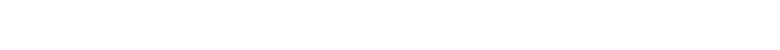This in turn introduces several new terms:Here, we’re particularly interested in k, the turbulent kinetic energy. Making a mathematical model for k gives us a way of calculating the generation (and the associated loss) of kinetic energy in a flow based on the other fluid properties. Not only that, but this also means that we can create an associated transport model for the properties of turbulence, allowing us to treat it just like the other properties such as mass, momentum and energy.

There are two main approaches to this kind of turbulence modelling, with the difference being how the dissipation of the turbulence is calculated. In the first, the “k-ε” (K-epsilon) model, ε is the rate at which k is converted into thermal energy. In the second, the “k-ω” (K-omega) model, ω is the “specific” dissipation rate per unit volume and time, proportional to the ratio of ε and k.

It might seem like we’ve included a lot of incredibly specific detail to get to this point, but it’s for a reason. These models, known as the “2-equation” RANS models, are by far the most common approach to CFD.

For the sake of completeness, sitting somewhere between the DNS approach and RANS models is Large-Eddy Simulation (LES), in which the larger turbulent lengthscales are resolved while the smaller lengthscales are filtered out with their contribution to the flow modelled in a similar fashion to the above. Each approach has their strengths and weaknesses, and it is up to the user to determine which is most appropriate.

In the case of the RANS models, one of these weaknesses is a further challenge; a model that works well in one flow environment might be inaccurate in another. As a basic example, the k-ε model is most accurate in the free-stream of the flow, while k-ω is the most accurate for flows close to boundaries. In many real fluid applications (such as flow through a pipe or over an aircraft wing), these different environments might be part of the same problem! Increasing accuracy in one flow type using a modelling approach has typically come at the cost of accuracy in another.

In some cases, the impact of these differences can be relatively trivial. In others, small inaccuracies can cause major problems. Some help is at hand; more recent techniques such as the k-ω Shear Stress Transport (SST) model can effectively switch between the behaviour of the different models depending on local flow conditions, but there’s still a considerable amount of expertise and physical data required to ensure that the results are valid and accurate.

Turbulence also isn’t the only problem that requires different methods and techniques. Compressible flows, and shockwaves in particular, also pose difficulties that require a more specialised treatment of flux transport that takes into account the flow direction.

Overcoming these problems currently can’t be done in a unified way, so rather than one single approach to CFD, what we’re left with is a fractured landscape of techniques and models, each with their own strengths and weaknesses. Awareness of this vast range of limitations and assumptions is currently critical for the successful (i.e useful, safe) application of CFD to real problems, which means that the number of people who can make use of CFD is considerably lower than the number of people and organisations who could benefit from it.

To sum up this problem in the words of NASA:

“At the moment, CFD is not yet sufficiently predictive and automated to be used in critical/relevant engineering decisions by the non-expert user, particularly in situations where separated flows are present.” — NASA CFD Vision 2030 study

### CFD in brief: A summary

We’ve covered a lot in this article, from the basic idea of making an approximate solution through to some of the big practical problems that current CFD methods encounter. Here’s a summary of what we’ve said.

• Some problems are complex enough that they can’t be solved through the use of analytic mathematical methods; we have to make numerical approximations.

• Fluid dynamics is one of these problems.

• Making approximations in fluid dynamics means taking the mathematical description of fluid behaviour, the Navier-Stokes equations, and converting them into a form that can be solved with computers

• The approximate method means that the individual calculations are much simpler, but now we have to perform them repeatedly, over potentially millions of individual elements that describe a region of flow.

• In practice there are many different ways of setting up these equations, which depend on the assumptions we make

• CFD is therefore all about trade-offs; do we need the simulation to be 3D or will 2D do? Do we want full resolution of the turbulence or will a simplified engineering model do?

• Answering these questions, selecting the best methods, and understanding what this means for the accuracy of the simulation requires specialist knowledge. Even the ability to construct a good quality mesh is highly dependent on understanding fluid dynamics and the methods you’re going to apply.

• CFD is “expensive” in all respects; time, money and energy.

The major reasons for this expense are:

• Timestepping: Outside of cases where the flow is in a steady-state, timestepping requires that we repeatedly solve problems that we aren’t necessarily interested in to reach the solutions that we are.

• Scale: The need to break fluids up into many smaller elements means that large simulations require incredible amounts of processing power.

• Complexity: Our current methods for performing CFD mostly rely on translating the differential equations that model fluid behaviour into something computers can work with. Because fluids aren’t simple, all but the most basic flows require us to use very expensive methods or a range of different modelling techniques.

We’ve said a lot in this article and yet barely scratched the surface of CFD. We’ve described some of the most common methods used for the most common fluid types, but there’s a huge range of problems where further modification is required. This includes things like non-Newtonian fluids (where the Boussinesq hypothesis can’t be applied), multi-phase flows, chemically reacting flows and many more. For all that this article has contained a fair amount of technical complexity, the sheer scope of CFD is vastly more complex still.

So wouldn’t it be nice if there was another way of doing CFD, one that came from a completely different direction? One that could do away with some of the core difficulties and expenses?

In our next article, we explain what this approach might be. Best of all, the techniques used in this approach are also compatible with our next-generation optical processing hardware, and so we also demonstrate the first application of optical computing to this method.

For now, if you’d like to know more about our objectives and methods in this area or would like to suggest another application for our technology, you can always contact us here.